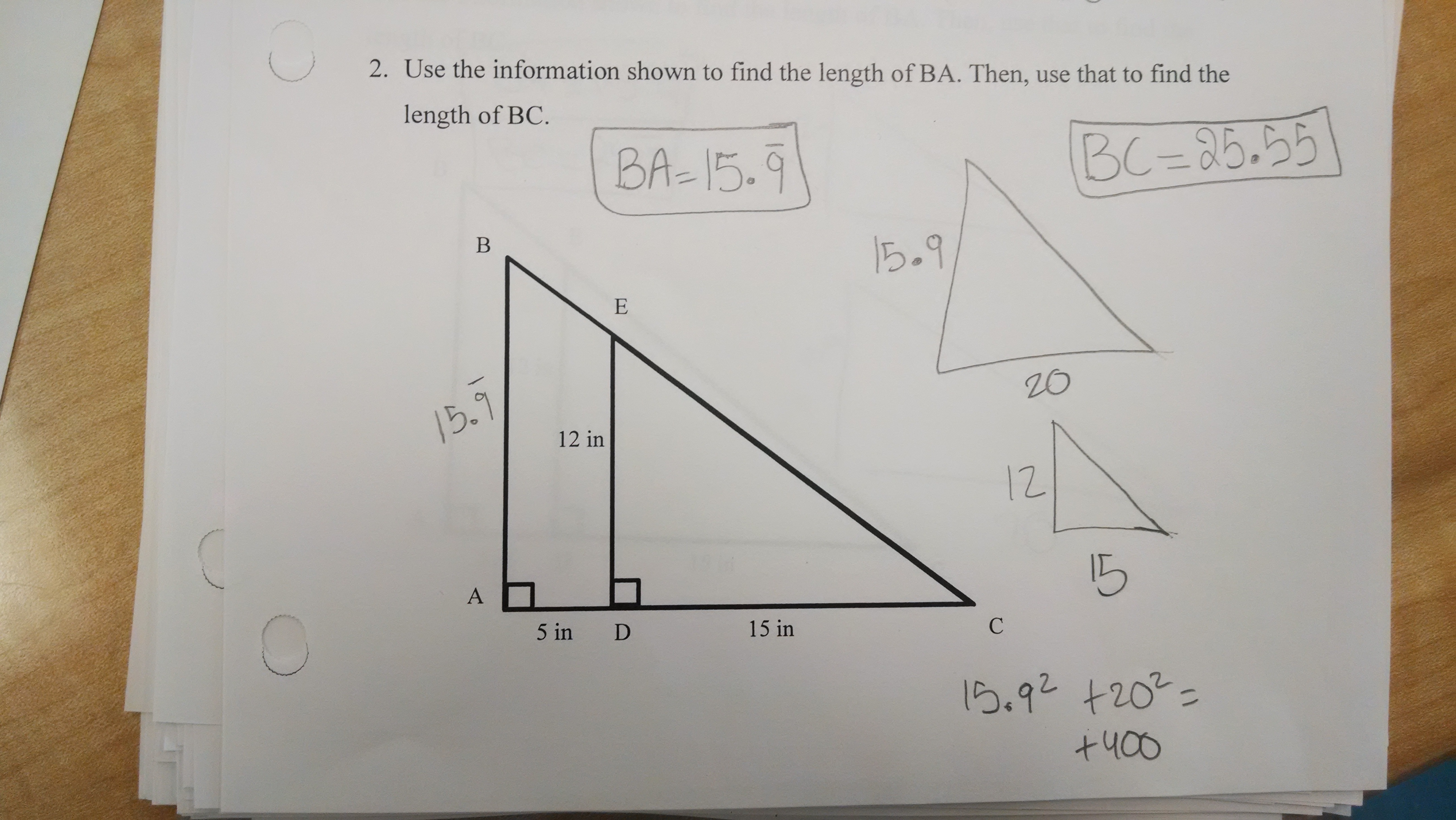Categories

# Decimal Misconceptions? Meet similar triangles.A note from the submitter:

Along the lines of one I sent you awhile back. This is one of my best students, and several other students gave answers with similar misconceptions. I pretty much ignored it last time it came up, thinking that it was an anomaly, but I think it’s a significant hole in my students’ understanding. Students were using calculators today.

What’s going on here in the student work? What’s the connection to the earlier post?

## 4 replies on “Decimal Misconceptions? Meet similar triangles.”

Curious about the use of the repeating decimal notation for the side but leaving it off for the Pythagorean Theorem. The Pythag part would not behave well with a repeating decimal, so I see leaving that off. I could see one of my snarky students substituting the 15.9999999999 for 16 since we have that debate, but they’d resort to the 16 for Pythag (at least I hope they would)
Since there is no work shown for the length of AB I’d probably start there by probing for problem-solving strategies there.

(Length BA)/20 = 12/15 (similar triangles)
How a calculator gets 15.999999999999999 for this I cannot see.

The 15.999… is presumably just peculiar calculator behavior and not stopping to simplify. The 25.55 is Sqrt[20^2+15.9^2], so the student only copied one 9 when entering the next value. Grading this one would be frustrating, since the first answer is right but wrong-headed and the second is very close to right.

By the way, I just stumbled into your blog and find it addictive. How far back can I answer these teasers before it becomes annoying?

i tend to think that the student did this:

20/ 15 =1.3333333 repeating.
12 * 1.33333 (pick a random amt of 3s to key into the calc) = 15.99996 or so, interpret as 15.999999 repeating.

then in doing pythagoras, the student didn’t know what to do with 15.9 repeating, so the student simply used 15.9, as if you do pythagoras with 15.9 and 20, you do indeed get 25.55

to me it seems like the student is unsure of how to handle repeating decimals. it might also help to work with the student on how the calculator holds onto an answer — there is no need to rekey 1.33333. the student may not understand that they can do:
20/15 = 1.3333333
ans * 12 = 16